Ch 9. Columns Multimedia Engineering Mechanics BasicColumns FixedColumns EccentricLoads
 Chapter 1. Stress/Strain 2. Torsion 3. Beam Shr/Moment 4. Beam Stresses 5. Beam Deflections 6. Beam-Advanced 7. Stress Analysis 8. Strain Analysis 9. Columns Appendix Basic Math Units Basic Equations Sections Material Properties Structural Shapes Beam Equations Search eBooks Dynamics Fluids Math Mechanics Statics Thermodynamics Author(s): Kurt Gramoll ©Kurt GramollMECHANICS - CASE STUDY SOLUTION Generally, columns are loaded at their center so that there is no eccentric forces. However, due to construction problems or design reasons some columns must support loads that are off set from their center, which are called eccentric loads. In this problem, a wind generator system is attached to vertical 6 in pole at its edge. Thus, the weight of the generator, 5 kips, is off center by 3 in. This eccentric load will cause the pole to bend and induce added bending stresses. This problem can be solved using the standard secant formula. Total Stress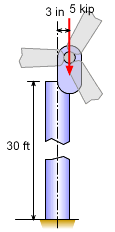Wind Generator Column with Off Center Load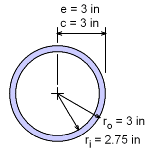Pole Cross Section The secant formula provides a method to determined the stress in a column with an eccentric load. The standard form of the formula is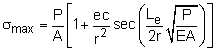Since the load P is given, 5 kips, the equation is easy to use. The other parameters are,      A = (ro2 - ri2)π = (32 - 2.752) π = 4.515 in2      e = 3 in (eccentric distance)      c = 3 in (furthest distance to outside edge)      I = (ro4 - ri4)π/4 = (32 - 2.752) π/4 = 18.70 in4      r = (I/A)0.5 = (18.70/4.515)0.5 = 2.035 in      E = 29,000 ksi The column height, L, needs to be converted to effective length since this is not a pin-pin column. For a fixed-free column, the effective length is      Le = 2L = 2(30 ft) = 60(12) in = 720 in Substituting these values into the secant formula gives,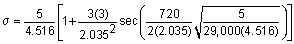= 1.107 [1 + 2.173 sec(1.0931)]         = 1.107 [1 + 2.173 (2.175)] The final stress which includes both compression and bending stress is      σ = 6.339 ksi The failure stress of the material is 36 ksi, but there is a factor of safety of 3. Thus the allowable is only 12 ksi. However, this the actual stress, 6.34 ksi is less than the allowable, so the design is still good. Maximum Load From the calculations above, a load of 5 kips will only produce a stress of 6.34 ksi which is below the allowable or 12 ksi. So how high can the load go before the stress hits 12 ksi? The secant formula can be used again, but this time the unknown will be P, not σ. Substituting the values in gives,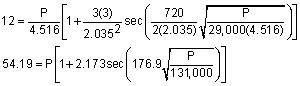The variable P cannot be solved using just algebra. A numerical method needs to be used or even trail and error. One simple and effective method to solve any single equation is to graph the error as a function of the variable. This is done by first rearranging the equation as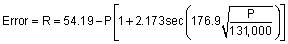The solution for P is when R goes to zero. This equation is shown in the diagram on the left.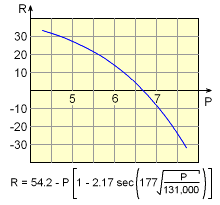Solution Curve for Load P The variable P cannot be solved using just algebra. A numerical method needs to be used or even trail and error. One simple and effective method to solve any single equation is to graph the error as a function of the variable. This is done by first rearranging the equation asThe solution for P is when R goes to zero. This equation is shown in the diagram on the left. The accuracy can be improved by narrowing the equation range. Using this method, the final solution is approximately       P = 6.660 kips

Practice Homework and Test problems now available in the 'Eng Mechanics' mobile app
Includes over 400 problems with complete detailed solutions.
Available now at the Google Play Store and Apple App Store.